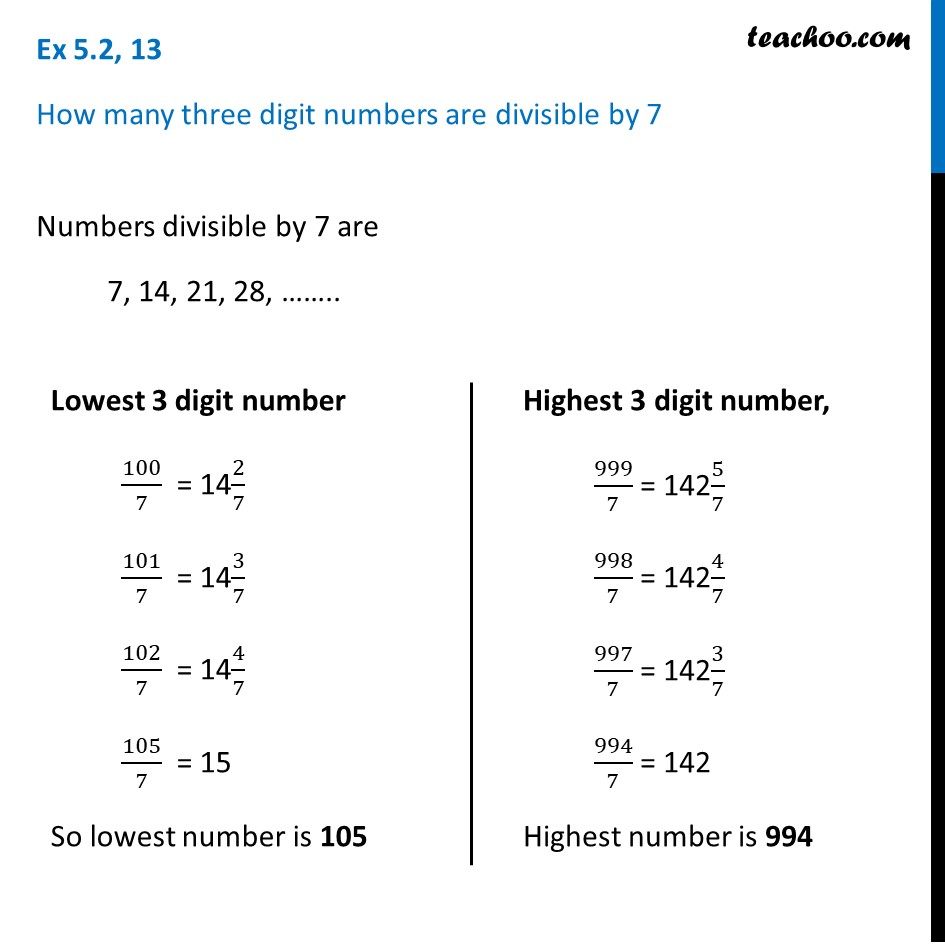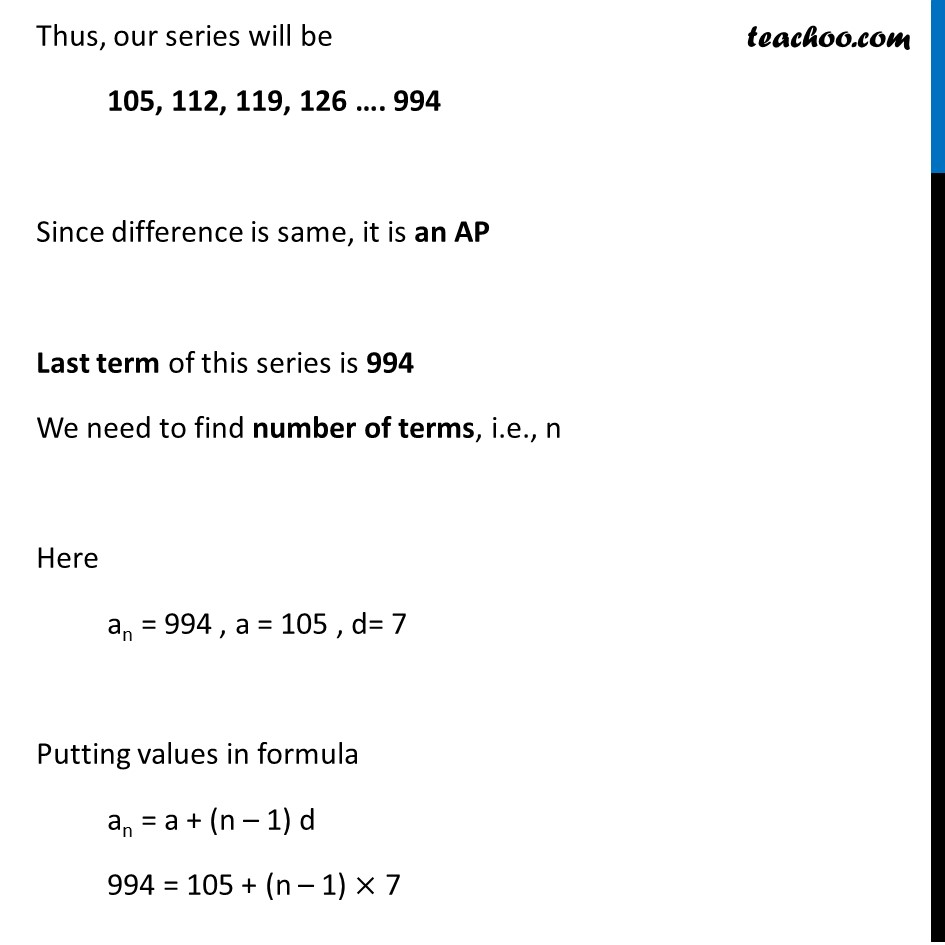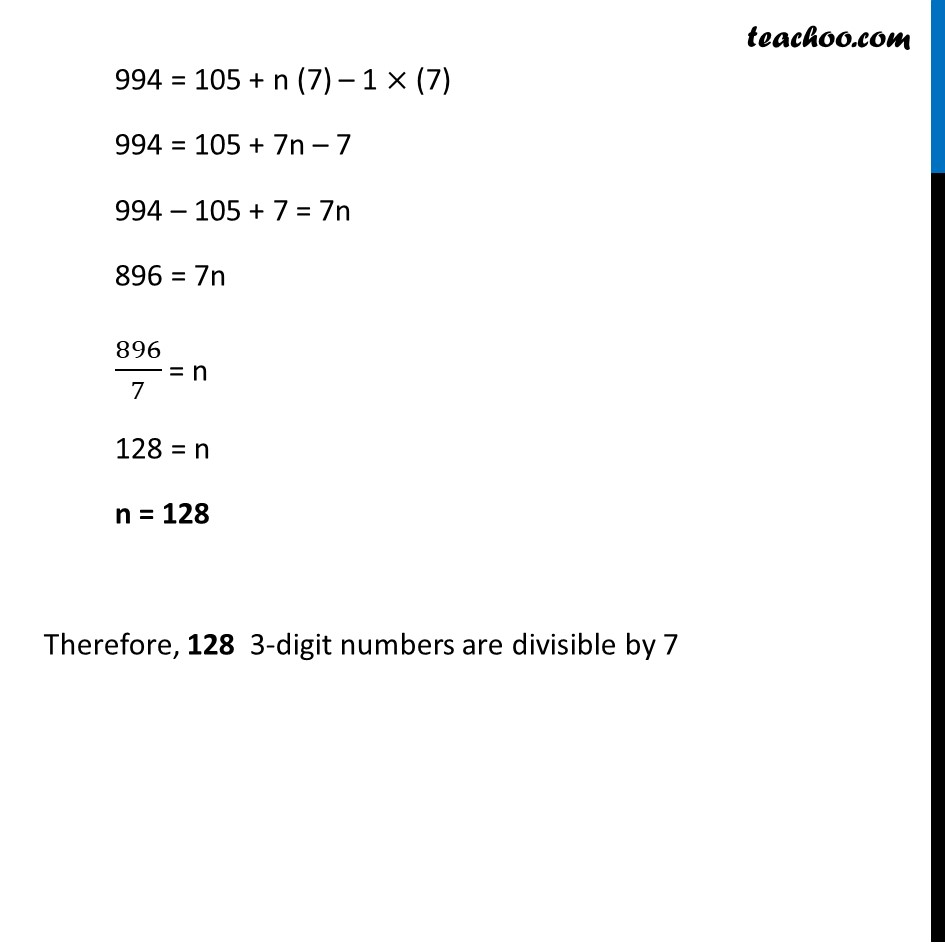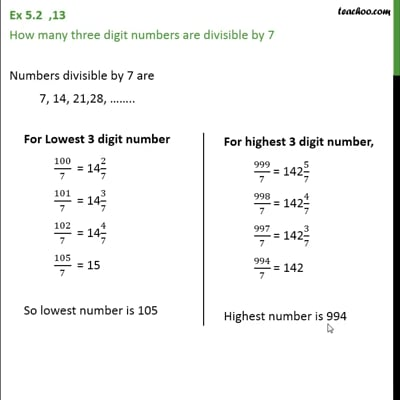Ex 5.2

Chapter 5 Class 10 Arithmetic Progressions
Serial order wiseThis video is only available for Teachoo black users

Learn in your speed, with individual attention - Teachoo Maths 1-on-1 Class

### Transcript

Ex 5.2, 13 How many three digit numbers are divisible by 7 Numbers divisible by 7 are 7, 14, 21, 28, …….. Lowest 3 digit number 100/7 = 142/7 101/7 = 143/7 102/7 = 144/7 105/7 = 15 So lowest number is 105 Highest 3 digit number, 999/7 = 1425/7 998/7 = 1424/7 997/7 = 1423/7 994/7 = 142 Highest number is 994 Thus, our series will be 105, 112, 119, 126 …. 994 Since difference is same, it is an AP Last term of this series is 994 We need to find number of terms, i.e., n Here an = 994 , a = 105 , d= 7 Putting values in formula an = a + (n – 1) d 994 = 105 + (n – 1) × 7 994 = 105 + n (7) – 1 × (7) 994 = 105 + 7n – 7 994 – 105 + 7 = 7n 896 = 7n 896/7 = n 128 = n n = 128 Therefore, 128 3-digit numbers are divisible by 7